# Competition Level Test: Straight Lines- 2

## 30 Questions MCQ Test Mathematics For JEE | Competition Level Test: Straight Lines- 2

Description
Attempt Competition Level Test: Straight Lines- 2 | 30 questions in 60 minutes | Mock test for JEE preparation | Free important questions MCQ to study Mathematics For JEE for JEE Exam | Download free PDF with solutions
QUESTION: 1

### Angles made with the x - axis by two lines drawn through the point (1, 2) and cutting the line x + y = 4 at a distance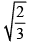from the point (1,2) are

Solution:

Let P≡(1,2)
Using the parametric form ,  the coordinates of the point at a distance of (2/3)^1/2 is given by,
(1+(2/3)^1/2cosθ, 2+(2/3)^1/2 sinθ)
This point lies on the line  x+y=4
Hence,
3+(2/3)^1/2(sinθ+cosθ)=4
On squaring and simplifying, we get
sin^2θ+cos^2θ+2sinθcosθ=3/2
1+sin2θ=3/2
sin2θ=1/2
The possible values of θ are π/12 and 5π/12

QUESTION: 2

### Consider a family of straight lines (x + y) + λ(2x - y + 1) = 0. Find the equation of the straight line belonging to this family that is farthest from (1, -3)

Solution:

Explanation : Consider a family of straight lines.

(x+y)+λ(2x−y+1)=0

This family consists of two lines:

x+y=0   and  2x−y+1=0

Add the two equations to get,

3x+1 = 0

3x = −1

x= −1/3

Put this value in x+y=0

−1/3 + y = 0

y = 1/3

So we have (x,y) = A(−1/3, 1/3)

Let the given point be B(1,−3)

Note that the required line is perpendicular to line AB.

The slope of the line AB is,

m1 = (−3−1/3)/(1+1/3)

= -10/4

m1 = - 5/2

So the slope of the required line is,   m2 = −1/m1

= −1/(−5/2)

= 2/5

Use point slope form to find the required equation of line.

y − y1 = m2(x − x1)

(y−1/3) = 2/5(x+1/3)

(3y−1) = 2/5(3x+1)

5(3y−1) = 2(3x+1)

15y−5=6x+2

15y−6x−7=0

So the required equation of line is,

15y − 6x − 7 = 0

QUESTION: 3

### One side of an equilateral triangle is 3x+4y=7 and its vertex is (1,2). Then the length of the side of the triangle is

Solution:

Length of the perpendicular from
(x1,y1) to the line ax+by+c=0 is
p= (ax1+by1+c)/[(a)2 + (b)2]1/2|
Let a be the length of each side of the equilateral triangle.
The length of the perpendicular p from (1,2) on 3x+4y−9=0
⇒p= |(3+8−7)/[(3)2 + (4)2]1/2|
= 4/5
sin60=p/a
⇒ [(3)1/2]/2 = [(4/5)]/a
⇒  a = (2)/(3)1/2 = 4/5
a = [8/5(3)1/2]

QUESTION: 4

Equation of the line through the point of intersection of the lines 3x+2y+4=0 and 2x+5y-1=0 whose distance from (2,-1) is 2

Solution:

Point of intersection (-2,1) and verification

QUESTION: 5

The acute angle bisector between the lines 3x-4y-5 = 0, 5x+12y-26 = 0 is

Solution:

a1a2 +b1b2  = -32 < 0, c1c2 = 130 > 0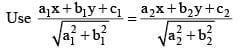QUESTION: 6

Find the equation of the bisector of the angle between the lines x+2y–11 = 0, 3x–6y–5 = 0 which contains the point (1,–3)

Solution:

Using formula given in synopsis

QUESTION: 7

A straight line which make equal intercepts on +ve x and y axes and which is at a distance '1' unit from the origin intersects the straight line y = 2x + 3 + √2 at (x0, y0) then 2x0 + y0

Solution:

Equation of the straight line having equal intercepts is x + y = k and proceed.

y0 = 2x + 3 + √2
x0 + y0 = √2.............(1)
x0 + 2x0 + 3 + √2 = √2
x0 = -1
Put the value of x in eq (1)
y0 = √2 - 1
2x0 + y0 = √2 - 1

QUESTION: 8

If P (1+t/√2, 2+t/√2) be any point on a line, then the range of the values of t for which the point p lies between the parallel lines x+2y = 1 and 2x + 4y = 15 is

Solution:

Given P (1 + t/√2, 2 + t/√2) lies on x + 2y = 1

Then 1 + t/√2 + 2 (2 + t/√2) = 1

⇒  1 + t/√2 + 4 + 2t/√2 = 1

⇒ 5 + 3t/√2 = 1

⇒ 3t/√2 = -4

⇒ 3t = -4√2

⇒ t = -4√2/3

Also P lies on 2x + 4y = 15

Then, 2 (1 + t/√2) + 4 (2 + t/√2) = 15

⇒ 2 + 2t/√2 + 8 + 4t/√2 = 15

⇒ 10 + 6t/√2 = 15

⇒ 6t/√2 = 5

⇒ 6t = 5√2

⇒ t = 5√2/6

Hence, the range will be -4√2/3 < t < 5√2/6

QUESTION: 9

Locus of point of intersection of the perpendicular lines one belonging to (x + y – 2) + λ(2x + 3y – 5) = 0 and other to (2x + y – 11) + λ(x + 2y – 13) = 0 is a

Solution:

By observation, both of them are non parallel lines. Locus of non parallel lines is a circle.

QUESTION: 10

A non-trival solution of the system of equations x + λy + 2z = 0 , 2x + λz = 0 , 2λx - 2y + 3z = 0 is given by x : y : z =

Solution:

Use sine rule that is a = 2R sinA,b = 2R sinB, c = 2R sinC.

QUESTION: 11

The number of values of k for which the linear equations 4x + ky + 2z = 0 , kx + 4y + z = 0 , 2x + 2y + z = 0 posses a non zero solution is

Solution:

Since, equation has non-zero solution.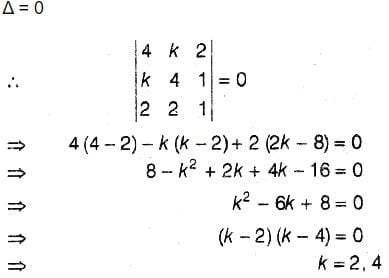Hence, number of values of k is two.

QUESTION: 12

The locus of the image of the point (2, 3) in the line (x - 2y + 3) + λ (2x - 3y + 4) = 0 (λ ∈ R) is

Solution:

A (2, 3) , B (1, 2) , P (x, y)
AB = BP

QUESTION: 13

A straight line L with negative slope passes through the point (8, 2) and cuts the positive co-ordinate axes at points P and Q.  As L varies, the absolute minimum value of OP + OQ (O being origin) is

Solution:

y - 2 = -m (x - 8) , where m >0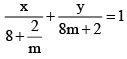QUESTION: 14

When the origin is shifted to a point P, the point (2, 0) is transformed to (0, 4) then the coordinates of P are

Solution:

(x, y) = (2, 0), (X, Y) = (0, 4)
(x – X, y – Y) = (2, -4)
New origin, (x - X, y - Y)

QUESTION: 15

If the transformed equation of a curve when the origin is translated to (1, 1) is X2 + Y2 + 2X – Y + 2 = 0 then the original equation of the curve is

Solution:

After shift of origin, New coordinates: X = x - 1, Y = y - 1
The equation X2 + Y2 + 2X – Y + 2 = 0, w.r.t to original origin:
(x-1)2 + (y-1)2 + 2(x-1) – (y-1) + 2 = 0
x2 + 1 - 2x + y2 + 1 - 2y + 2x - 2 – y + 1 + 2 = 0
x2 + y2 - 3y + 3 = 0

QUESTION: 16

In order to make the first degree terms missing in the equation 2x2 + 7y2 + 8x – 14y + 15 = 0, the origin should be shifted to the point

Solution:

a = 2, b = 7, g = 4, f = -7, c = 15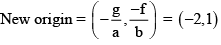QUESTION: 17

The distance of any point (x, y) from the origin is defined as d = max {| x |, | y |} , then the distance of the common point for the family of lines x(1 + λ) + λy + 2 + λ = 0  (λ being parameter) from origin is

Solution:

Foxed point P ≡ (-2,1)
Distance of P from origin = 2.

QUESTION: 18

If the point P(α2, α) lies in the region corresponding to the acute angle between the lines x - 3y = 0 and x - 5y = 0 then

Solution:

P lies in the acute angle
⇒ α2 - 3α > 0 and α2 - 5α < 0
⇒ α ∈ (-∞, 0) ∪ (3,∞) and α ∈ (0,5)
∴ α ∈ (3,5)

QUESTION: 19

Let ax + by + c = 0 be a variable straight line, where a, b and c are 1st, 3rd and 7th terms of some increasing A. P. Then the variable straight line always passes through a fixed point which lies on

Solution: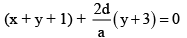∴ Fixed point ≡ (2, -3) which lies on x2 + y2 = 13

QUESTION: 20

Give the family f lines, a(2x + y + 4) + b(x – 2y – 3) = 0.amongteh lines of the family, the number of lines situated at a distance of √10 from the point M(2, -3) is

Solution:

The point of intersection of the two lines are (-1,- 2).
Distance PM = √10
Hence the required line is one which passes through (-1, -2) and is perpendicular to P.M.

QUESTION: 21

Angle between the lines x2 + 2xy sec α + y2 = 0 is

Solution:

(x2 + 2xy secα + y2) = 0
(a=1) (h = secα) (b=1)
tanθ = 2[(h2- ab)]½/(a+b)
tanθ = 2[((secα)^2 - 1)]½/(1+1)
tanθ  = [(tan2)α]½
tanθ = tanα
⇒ θ = α

QUESTION: 22

If θ is the acute angle between the pair of liens x2 + 3xy – 4y2 = 0 then sin θ =

Solution:

x2 + 3xy - 4y2 = 0
a = 1, b=-4
h = 3/2
tanθ = 2[(h2 - ab)½]/a+b
= 2[(9/4 - 1(-4))½]/1+(-4)
= 2[(9/4 + 4)½]/(-3)
= [2(5/2)]/(-3)
tanθ = 5/3 which is (perpendicular/base)
sinθ = 5/[(5)2 + (3)2]
sinθ = 5/(34)½

QUESTION: 23

The product of perpendiculars from (k, k) to the pair of lines x2 + 4xy + 3y2 = 0 is 4/√5 then k is

Solution:

Explanation : x2 + 4xy + 3y2 = 0

x2 + xy + 3xy + 3y2 = 0

x(x+y) + 3y(x+y) = 0

(x+3y) (x+y) = 0

Points (k,k)

d1 = |(k+k)/(2)1/2|

2k/(2)1/2

d2 = |(k+3k)/(1+9)1/2|

d2 = |4k/(10)1/2|

d1 * d2 = 4/(5)1/2

|(k+k)/(2)1/2| * |4k/(10)1/2| = 4/(5)1/2

|8k2/(20)1/2| = 4/(5)1/2

8k2/2(5)1/2 = 4/(5)1/2

k2 = +-1

QUESTION: 24

The value k such that 3x2 + 11xy + 10y2 + 7x + 13y + k = 0 represents a pair of straight lines is

Solution:

Δ = 0

QUESTION: 25

Angle between the pair of lines 2x2 – 7xy + 3y2 + 3x + y – 2 = 0

Solution:

Here, a=2,b=3 and h=−72
Let θ be the angle between the lines represented by 2x2−7xy+3y2=0. Then ,
tanθ=(2√49/4−6)/5=1
⇒ θ = 45.
⇒ π/4

QUESTION: 26

If the angle between the pair of lines 2x2 + λxy + 3y2 + 8x + 14y + 8 = 0 is (π/4), then the value of λ is

Solution:

2x2 + λxy + 3y2 + 8x + 14y + 8 = 0
ax2 + 2hxy + by2 + 2gx + 2fy + c = 0
Compare eq (1) and (2), we get
a =2, b = 3, 2h = λ
tanθ=(2√h2 - ab)/(a+b)
=> tan(π/4) = [(2√(λ/2)2 - (2)(3))/(2+3)]
=> 1 = [(2√((λ)2)/4 - 6]/5
=> 5 = [(2√((λ)2)/4 - 6]
=> 25 = [4((λ)2)/4 - 6]
=> 25 = (λ)2 - 24
=> (λ)2 = 49
=> λ = +-7

QUESTION: 27

If the distance between the pair of parallel lines x2 + 2xy + y2 – 8ax – 8ay – 9a2 = 0 is 25√2units. What is 'a'?

Solution:
QUESTION: 28

The equation to the pair of lines joining the origin to the points of intersection of y = x + 3 and 2x2 + 2y2 = 1 is

Solution: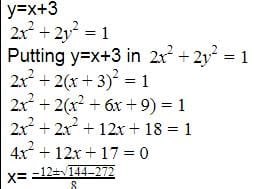Here x is a complex number. So circle and line does not intersect. So there is no solution

QUESTION: 29

If the lines ax + by + c = 0, bx + cy + a = 0 and cx + ay + b = 0 are concurrent then the point of concurrency is

Solution: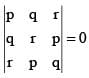(P + q + r) (p+ q+ r2 - pq - qr - rp) = 0

QUESTION: 30

If the lines 3x + 2y – 5 = 0, 2x – 5y + 3 = 0, 5x + by + c = 0 are concurrent then b + c =

Solution:

We know that concurrent lines have same intersection points.Then
∴ 3x+2y−5=0 --(1)
2x−5y+3=0 ---(2)
5x+by+c=0 ---(3)
From 1 & 2,
6x+4y−10=0
6x−15y+9=0
⇒19y−19=0
y=1 & x=1
So, substituting  x=1 & y=1, in equation (3),
5x+by+c=0
5+b+c = 0
∴ b+c = −5Use Code STAYHOME200 and get INR 200 additional OFF Use Coupon Code